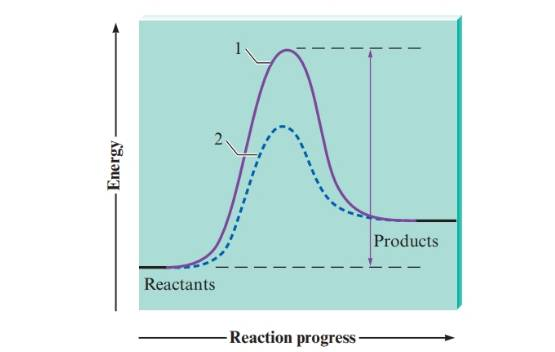Chapter 17, Problem 14ALQ### Introductory Chemistry: A Foundati...

9th Edition
Steven S. Zumdahl + 1 other
ISBN: 9781337399425

#### Solutions

Chapter
Section### Introductory Chemistry: A Foundati...

9th Edition
Steven S. Zumdahl + 1 other
ISBN: 9781337399425
Textbook Problem
5 views

# . Consider the figure below in answering the following questions.a. What does a catalyst do to a chemical reaction? b. Which of the pathways in the figure is the catalyzed reaction pathway? How do you know? c. What is represented by the double-headed arrow?

Interpretation Introduction

(a)

Interpretation:

The change occurs in the chemical reaction due to the presence of catalyst is to be identified.

Concept Introduction:

A catalyst is a substance that is added to a specific reaction to increase its rate of reaction. The concentration of catalyst remains same even after the completion of reaction that is why a catalyst is required in small quality for a reaction.

The activation energy of a reaction is the minimum energy required to precede the reaction.

Explanation

A catalyst is used increase the rate of reaction. The catalyst increases the rate of the reaction by lower the activation energy of the reaction. A small amount of catalyst is requi...

Interpretation Introduction

(b)

Interpretation:

The catalyzed reaction pathway in the given figure is to be identified.

Concept Introduction:

A catalyst is a substance that is added to a specific reaction to increase its rate of reaction. The concentration of catalyst remains same even after the completion of reaction that is why a catalyst is required in small quality for a reaction.

The activation energy of a reaction is the minimum energy required to precede the reaction.

Interpretation Introduction

(c)

Interpretation:

The quantity represented by double-head arrow is to be identified.

Concept Introduction:

A catalyst is a substance that is added to a specific reaction to increase its rate of reaction. The concentration of catalyst remains same even after the completion of reaction that is why a catalyst is required in small quality for a reaction.

The activation energy of a reaction is the minimum energy required to precede the reaction.

### Still sussing out bartleby?

Check out a sample textbook solution.

See a sample solution

#### The Solution to Your Study Problems

Bartleby provides explanations to thousands of textbook problems written by our experts, many with advanced degrees!

Get Started

#### Does the cell cycle refer to mitosis as well as meiosis?

Human Heredity: Principles and Issues (MindTap Course List)

#### What is the tragedy implicit in Hardins The Tragedy of the Commons?

Oceanography: An Invitation To Marine Science, Loose-leaf Versin

#### Complete the following table:

Foundations of Astronomy (MindTap Course List)